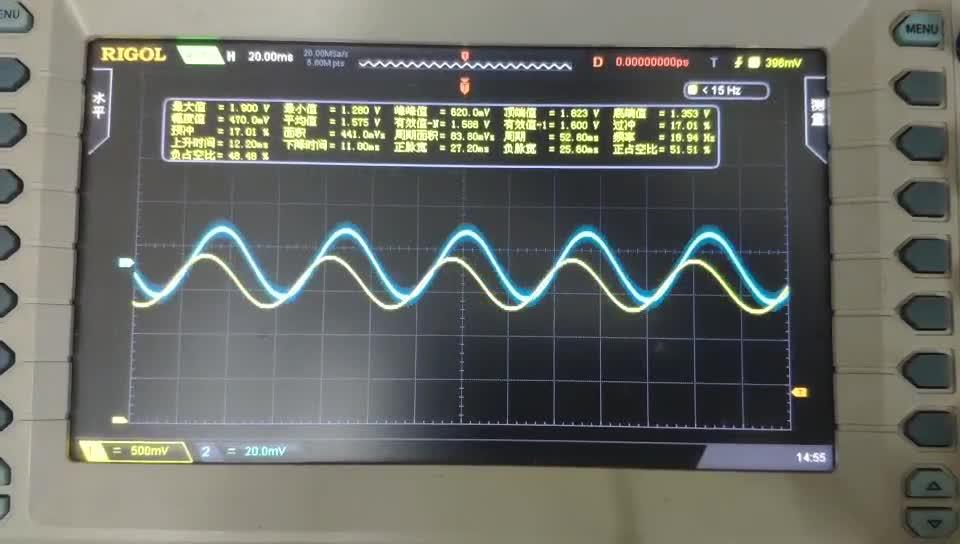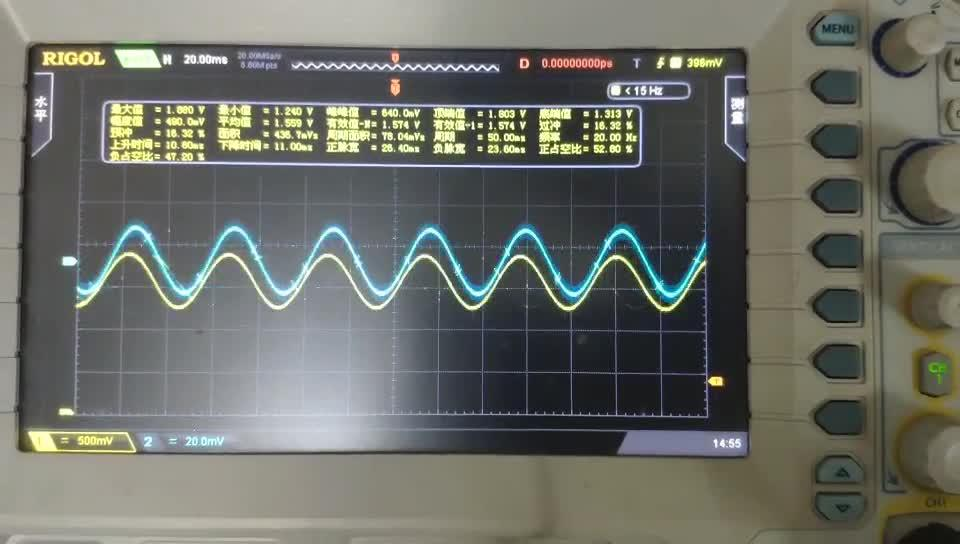## 2、滤波器定义

• 低通滤波器（Low-pass Filter，LPF）
• 高通滤波器（High-pass Filter，HPF）
• 带通滤波器（Band-pass Filter，BPF）
• 带阻滤波器（Band-rejection Filter，BRF）
• 全通滤波器（All-pass Filter，APF）

## 2.1、滤波器种类

### 2.1.1、低通滤波器

f0～f2频率之间，幅频特性平直，它可以使信号中低于f2的频率成分几乎不受衰减地通过，而高于f2的频率成分受到极大地衰减。

### 2.1.2、高通滤波器

与低通滤波相反，从频率f1～∞，其幅频特性平直。它使信号中高于f1的频率成分几乎不受衰减地通过，而低于f1的频率成分将受到极大地衰减。

### 2.1.3、带通滤波器

它的通频带在f1～f2之间。它使信号中高于f1而低于f2的频率成分可以不受衰减地通过，而其它成分受到衰减。

### 2.1.4、带阻滤波器

与带通滤波相反，阻带在频率f1～f2之间。它使信号中高于f1而低于f2的频率成分受到衰减，其余频率成分的信号几乎不受衰减地通过。

## 3.2、滤波器计算

### 3.2.2、二阶低通滤波器

为改善滤波效果，使时，信号衰减的更快，一般在上图所示的一阶低通滤波器的基础上再增加一级RC电路就构成二阶有源低通滤波器，如下图所示。

## 3.2、二阶滤波器计算

LPF：假设待计算滤波器Q=0.7（读者可以根据实际情况取值，此处仅以0.7为例设计）、f0=35Hz。

HPF：由于同类型LPF和HPF具有对偶性，实际计算按照LPF计算，电路中替换RC位置即可。

LPF：R1=R2=18.19kΩ，实际取值R1=R2=18kΩ

HPF：R1=R2=42.46 kΩ，R1=R2=43kΩ

LPF：R1=14.784kΩ，R2= 23.6548‬ kΩ，实际取值R1=15 kΩ、R2=24kΩ

HPF：R1= 34.499 kΩ，R2= 55.198 kΩ，实际取值R1=33 kΩ、R2=56kΩ

## 5.2、PCB设计：

PCB部分根据实际生产的需求制作了两种拼版文件：V-cut和邮票孔，此部分可以直接使用，读者也可以实际动手操作一遍，此处使用到高级粘贴功能，具体操作此处不再赘述没有兴趣的读者可以自行了解，另外在做V-cut拼版时需要注意各家板厂V-cut使用钻头的直径，实际拼板中需要根据V-cut钻头的直径预留两个相邻板间的间距，此处按照默认0.4mm设计。

f=12Hz时：

f=20Hz时

f=60Hz时：

## Matlab 绘制bode图代码

%有源二阶模拟带通滤波器

%LPF 传递函数计算 f0=35Hz C = 1uF，R = R=4.549kΩ g1=k3/(s2+k1*s1+k2)

c1 = 1e-6;

r1 = 4549;

%HPF 传递函数计算 f0=15Hz C = 1uF，R = R=4.549kΩ g2=k6*s2/(s2+k4*s1+k5)

c2 = 1e-6;

r2 = 10615;

for q=0.1:0.2:3

%LPF

Avp1 = 3-(1/q);

%R1 = 2*r1*Avp1/(Avp1-1);

%R2 = 2*r1*Avp1;

k1 = (3-Avp1)/(c1*r1);

k2 = 1/(c1*c1*r1*r1);

k3 = Avp1/(c1*c1*r1*r1);

num1=[k3]; %传递函数分子

den1=[1 k1 k2]; %传递函数分母式为：s2+k1s+k2

G1=tf(num1,den1);

%HPF

Avp2 = 3-(1/q);

%R1 = 2*r2*Avp2/(Avp2-1);

%R2 = 2*r2*Avp2;

k4 = (3-Avp2)/(c2*r2);

k5 = 1/(c2*c2*r2*r2);

k6 = Avp2;

num2=[k6 0 0]; %传递函数分子，此处为s2需要特别注意

den2=[1 k4 k5]; %传递函数分母格，式为：s2+k4s+k5

G2=tf(num2,den2);

p=bodeoptions;

p.FreqUnits='Hz';

p.Grid= 'on';

[num,den] = series(num1,den1,num2,den2); %计算串联传递函数，串联传递函数需要相乘

printsys(num,den) %显示串联后的总传递函数

hold on;

bode(num,den,p); %绘制波特图

% hold on;

% bode(G1,p);

% hold on;

% bode(G2,p);

End

legend('0.1','0.3','0.5','0.7','0.9','1.1','1.3','1.5','1.7','1.9','2.1','2.3','2.5','2.7','2.9');

title('有源二阶模拟带通滤波器相频特性'); %标题https://www.zhihu.com/video/1188012220288667648https://www.zhihu.com/video/1188012458559549440